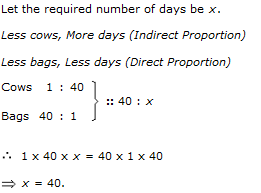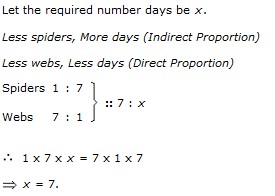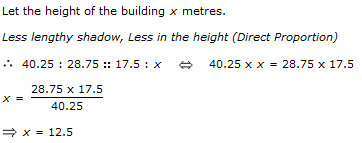6.

If a quarter kg of potato costs 60 yen, how many paise will 200 gm cost?

 A. yen 48 B. yen 54 C. yen 56 D. yen 72
Explanation: Let the required weight be x kg.

Less weight, Less cost (Direct Proportion)

250 : 200 :: 60 : x 250 x x = (200 x 60)

x= (200 x 60)/250

x = 48

7.

In a dairy farm, 40 cows eat 40 bags of husk in 40 days. In how many days one cow will eat one bag of husk?

 A. 1 B. 1/40 C. 40 D. 80
Explanation:8.

A wheel that has 6 cogs is meshed with a larger wheel of 14 cogs. When the smaller wheel has made 21 revolutions, then the number of revolutions mad by the larger wheel is:

 A. 4 B. 9 C. 12 D. 49
Explanation: Let the required number of revolutions made by larger wheel be x.

Then, More cogs, Less revolutions (Indirect Proportion)

14 : 6 :: 21 : x <-> 14 x x = 6 x 21

x = (6 x 21)/14

x = 9

9.

If 7 spiders make 7 webs in 7 days, then 1 spider will make 1 web in how many days?

 A. 1 B. 7/2 C. 7 D. 49
Explanation: